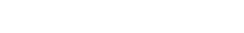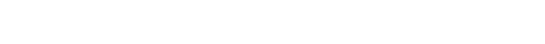## Kenya Certificate Of Secondary Education (KCSE 2008) Chemistry Paper 2

1.  Biogas is a mixture of mainly carbon (IV) oxide and methane.
1. Give a reason why biogas can be used as fuel. (1 mark)
2. Other than fractional distillation, describe a method that can be used to determine the percentage of the methane in biogas. (3 marks)

2. A sample of gas containing 35.2% by the mass of methane. A mass of biogas contains 5.0 kg of the gas.
Calculate the;
1. Number of moles of methane in the cylinder. (molar mass of methane = 16) (2 marks)
2. Total volume of carbon (IV) oxide produced by the combustion of methane in the cylinder. (Molar gas volume = 24.0 dm-3at room temperature and pressure.) (2 marks)

3. Carbon (IV) oxide, methane, nitrogen (I) oxide and trichlorofluoromethane are green-house gases.
1. State one effect of an increased level of the gases to the environment. (1 mark)
2. Give one source from which each of the following gases is released into the environment;
1. Nitrogen (I) oxide
2. Trichlorofluoromethane. (1 mark)

1. Write an equation to show the effect of heat on the nitrate of;
1. Potassium (1 mark)
2. Silver (1 mark)
2. The table below gives information about elements A1, A2, A3 and A4.1.  In which period of the periodic table is element A2? Give a reason. (2 marks)
2. Explain why the atomic radius of:
1. A1 is greater than that of A2; (2 marks)
2. A4 is greater than its ionic radius. (2 marks)
3. Select the element which is in the same group as A3. (1 mark)
4. Using dots (.) and crosses to represent outermost electrons, draw a diagram to show the bonding in the compound formed when A1 reacts with A4. (1 mark)

1. Describe the process by which nitrogen is obtained from air on a large scale. (4  marks)
2. Study the flow chart below and answer the questions that follow.1. Identify gas J. (1 mark)
2. Using oxidation numbers, show that ammonia is a reducing agent in step (VI). (2 marks)
3. Write an equation for the reaction that occurs in step (V). (1 mark)
4. Give one use of ammonium nitrate. (1 mark)

3. The table below shows the observations made when aqueous ammonia was added to cations of elements E, F and G until in excess.1. Select the cation that is likely to be Zn2+. (1mark)
2. Given that the formula of the cation of element E is E2+, write an equation between E2+(aq) and aqueous ammonia. (1 mark)

1. State the Le chatelier’s principle. (1 mark)
2. Carbon (II) oxide reacts with steam according to the equation;What would be the effect of increasing the pressure of the system at equilibrium? Explain. (2 marks)
3. When the reaction in (ii) above was carried out at lower temperature, the yields of hydrogen and carbon (IV) oxide increased.
What is the sign of ΔH for the reaction? Explain. (2 marks)

1. The table below gives the volumes of oxygen gas produced at different times when hydrogen peroxide decomposed in the presence of a catalyst.1. Name the catalyst used for this reaction. (1 mark)
2. On the grid provided, draw the graph of volume of oxygen gas produced (Vertical axis) against time. (3 marks)3. Using the graph determine the rate of decomposition of hydrogen peroxide after 24 seconds. (2 marks)
4. Give a reason why the total volume of oxygen gas produced after 50 seconds remains constant. (1 mark)

1. Alkanes, alkenes and alkynes can be obtained from crude oil. Draw the structure of the second member of the alkyne homologous series. (1 mark)
2. Study the flow chart below and answer the questions that follow.1. State the conditions for the reaction in step I to occur. (1 mark)
2. Identify substance H. (1 mark)
3. Give:
1. One disadvantage of the continued use of substances such as J. (1 mark)
2. The name of the process that takes place in step III. (1 mark)
3. The name and formula of substance K. (2 marks)
Name ……………………………………………………….
Formula …………………………………………………
4. The relative molecular mass of J is 16, 800. Calculate the number of monomers that make up J. (2 marks)

3. The table below gives the formulae of four compounds, L, M, N and P.Giving a reason in each case, select the letter which represents a compound that:
1. Decolorises bromine in the absence of UV light. (2 marks)
2. Gives effervescence when reacted with aqueous sodium carbonate. (2  marks)

1. The diagram below represents a set up that can be used to electrolyse aqueous coper (II) sulphate.1. Describe how oxygen gas is produced during electrolysis. (2 marks)
2. Explain why copper electrodes are not suitable for electrolysis. (2 marks)

1. Impure copper is purified by electrolysis process.
1. Name one ore from which copper is obtained. (1 mark)
2. Write the equation for the reaction that occurs at the cathode during the purification of copper. (1 mark)
3. In an experiment to electroplate a copper spoon with silver, a current of 0.5A was passed for 18 minutes. Calculate the amount of silver deposited on the spoon. (1 F = 96, 500 coulombs, Ag =108).       (3 marks)
4. Give two reasons why some metals are electroplated. (2 marks)

1. Define the standard enthalpy of formation of  a substance. (1 mark)
2. Use the thermochemical equations below answer the questions that follow.1. Name two types of heat changes represented by H3. (2 marks)
2. Draw an energy level diagram for the reaction presented in equation 1. (3 marks)
3. Calculate the standard enthalpy of formation of ethane. (2 marks)
4. When a sample of ethane was burnt, the heat produced raised the temperature of 500g of water by 21.5K. (Specific heat capacity of water = 4.2 Jg-1K-).
Calculate the;
1. Heat change for the reaction. (2 marks)
2. Mass of ethane that was burnt. (Relative formula mass of ethane = 30)  (2 marks)